• 25. 10. 2019
• Ing. Jan Zedníček - Data & Finance
• 0

Most calculations in the area of financial mathematics are relatively easy – for example, to calculate an annuity payment, interest rate or target amount value with regular deposits. However, financial calculations can be quite complex in certain circumstances.

This is particularly true on the money and capital markets (bonds) or on some specifically agreed corporate loans.

## Problem of Counting the Number of Days per Year and the Price of Coupon Bonds

The coupon bond has, among other things, its nominal value (= face value), the coupon rate in % p.a. and payment frequency. From these parameters, we are able to calculate the coupon (how much we get paid on interest eg per year). It sounds simple, but unfortunately, it is not.

Accrued interest for Bonds and day count conventions

In bond trades, it is common to buy a bond from the issuer after the issue date (mid-year) or between two coupon payments. As a result, interest has accrued from the issue date to the purchase date. We will have to pay this accrued interest along with the nominal price to the issuer (or the previous bondholder).

When calculating accrued interest, day count conventions come into play.

Note: On the issue date (or the day where is a coupon payment) is accrued interest equal to 0! (see figure, Nominal value = 10000, coupon rate = 3%, annual payments.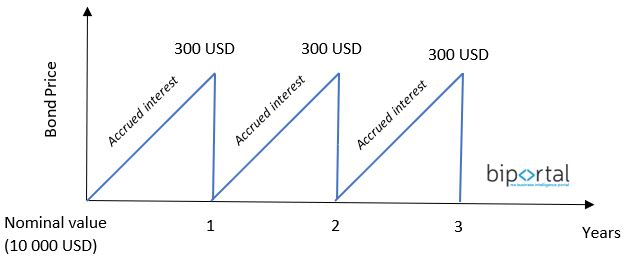For a more detailed explanation of the accrued interest, see the example:

• Issue date: 2016-02-01
• Purchase date (from the issuer or another subject): 2017-01-08
• First payment date: 2017-02-01
• Coupon rate: 3% p.a.
• Payment frequency: annual
• Face value (Nominal Value): \$ 10.000

If we buy the bond exactly at the time it was issued (or before that time or on the payout date), the price of the bond is equal to its nominal value (\$ 10,000). On 2017-02-01 we will receive a coupon equal to 3% * \$ 10,000 = \$ 300 (conclusion: In this situation, we do not count with Accrued interest at the time of bond purchase).

But that’s not the case, because we bought the bond later (2017-01-08). Now we have a problem. If we want to calculate the price of the bond, we must now include in our calculation a factor that takes into account how long the bond was held by the previous owner/issuer (ie from 2016-02-01 to 2017-01-08).

The calculation will be defined as follows:and therefore: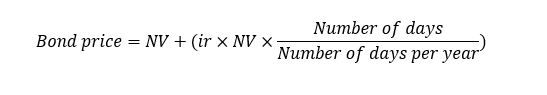Now there is a problem because we are unable to calculate the number of days between 2016-02-01 and 2017-01-08. Specifically, the number of days per year.

Moreover, 2016 was a leap year. To simplify interest calculations, various calculation methods have evolved over time. These are called day count conventions.

## Day Count Conventions – Definition and Explanation

As I mentioned, the reason for the methods is that we are not able to easily calculate the number of days between 2 dates in every situation.

The principle of all these methods is a direct determination of how days should be calculated regardless of the calendar days approach. This means that each method determines how many days it contains a year (regardless of reality) and how many days it has each month. Furthermore, some methods determine how to deal with the leap year, etc.

This, of course, is also important for capital market participants. It is not possible (or should not be) for the issuer, for example, to calculate other interests than the creditor. The interest calculation method must be determined equally for both.

Specific securities such as bonds or more complex loans (or other products) should always contain a rule on how interest is calculated – which day count calculation method should be used.

Let’s go back to the example from the previous chapter where we couldn’t calculate the price of a coupon bond – Let’s say this bond has a day count convention 30/360.

30/360 methods say that each month has 30 days and every year has 360 days. So if we go back to the previous chapter, where we were unable to calculate the Price of the bond, then we can do it now.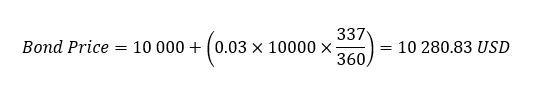Thus, the accrued interest is \$ 280.83, which has accrued since 2016-02-01

There are many methods of counting, but in principle, we can classify them into two categories

• 30/360 methods
• ACT methods (actual)

## 30/360 methods (Month = 30 days and Year = 360 days)

For 30/360 conventions, the number of days per month is equal to 30 and the number of days per year is 360. All methods based on the 30/360 principle have a common formula: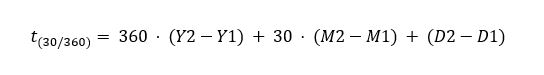where:

D…day -> D1 = start day, D2 = end day
M…month-> M1 = start month, M2 = end month
Y…year-> Y1 = start year, Y2 = end year

Individual 30/360 methods differ in that they solve different situations eg counting the first or last day between dates or how the model calculates if the first day is also the last day of the month and so on.

Conclusion: A common formula is established for these methods. Depending on the use of a particular method, we must check the conditions imposed by that method. These conditions adjust D1 or D2 when the condition is met.

### 30E/360 – European Method – German Method

A relatively simple method used mainly in Europe. It is also known as the German method. The number of days is calculated according to the following rules.

Conditions for D1 or D2

1. If the number of days is> 30, then => D1 or D2 to 30

### 30/360 US

The US method is almost the same as the 30E / 360 method with several differences. The method contains several additional situations that must be checked when using this method. If these situations occur, the method requires adjusting the number of days in a predetermined manner.

Conditions for D1 or D2

1. If D1 is the last day of February and at D2 is also the last day of February, then => D2 = 30
2. If D1 is the last day of February then => D1 = 30
3. If D2 is 31 and D1 is 30 or 31 then => D2 = 30.
4. If D1 is equal to 31 then => D1 = 30.

### 30E/360 ISDA – Eurobond Basis

It works the same way as the 30E / 360 method except that it contains 2 conditions for D1 and D2.

Conditions for D1 or D2

1. If D1 is the last day of the month then => D1 = 30.
2. If D2 is the last day of the month (except when D2 is equal to the maturity date and M2 is February) then => D2 = 30.

## ACT Methods – Based on the Actual Number of Calendar Days

These methods are based on the fact that either the number of days per month or the number of days per year is a real calendar number of days. There are a lot of ACT methods, the most commonly used are 3

• ACT/360 (French method)
• ACT/365 (English method)
• ACT/ACT ISDA

### ACT/360 – French Method

This method calculates the number of days in a month as the actual number of calendar days. The number of days per year is fixed at 360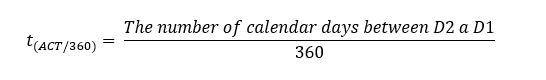where:

D…day -> D1 = start day, D2 = end day

### ACT/365 – English Method

This method calculates the number of days in a month as the actual number of calendar days. The number of days per year is fixed at 365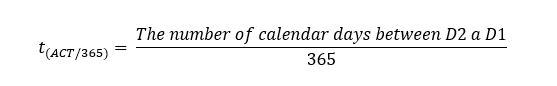where:

D…day -> D1 = start day, D2 = end day

### ACT/ACT ISDA

The number of days is calculated as the actual number of days between 2 dates. The number of days in a year is set at 365 or 366, depending on if it is a leap year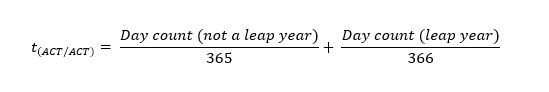Individual methods 30/360 and ACT were described using the source: Wikipedia

5/5 - (1 vote)### Ing. Jan Zedníček - Data & Finance

My name is Jan Zedníček and I work as a freelancer. I have been working as a freelancer for many companies for more than 10 years.
I used to work as a financial controller, analyst and manager at many different companies in field of banking and manufacturing. When I am not at work, I like playing volleyball, chess, doing a workout in the gym.